## Summary

A common effect people use in shaders in a fresnel effect. With a fresnel you can darken, lighten or color the outline of your objects, increasing the sense of depth.

For this tutorial we will make a surface shader, so if you follow it directly you should know the basics of surface shaders. You can find a explanation of them here. But you can also use a fresnel effect for unlit shaders, giving your objects some smoothness and tangibility without having to implement expensive lighting.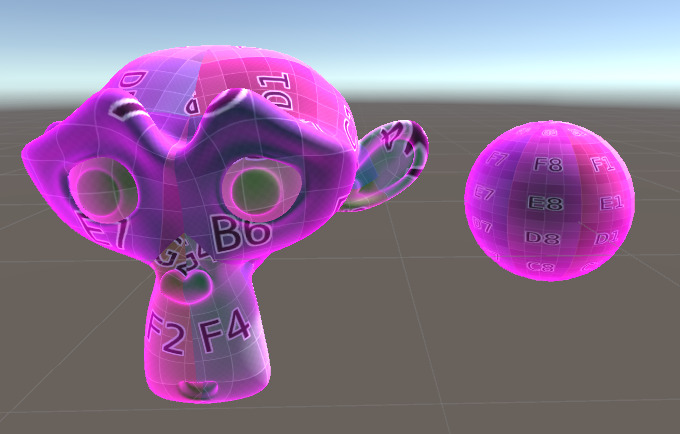## Highlighting one Side of the Model

We start with the surface shader modify it to show the fresnel. The fresnel uses the normals of the object to determine the intensity of the effect. To get the normals in worldspace in our shader, we add the worldNormal attribute to our input struct as well as the internal data macro. We won’t interact with the internal data at all, but unity needs it to generate the worldspace normal.

You can generate the worldspace normals in non-surface shaders with a simple matrix multiplication, it’s explained in my triplanar mapping tutorial.

``````//input struct which is automatically filled by unity
struct Input {
float2 uv_MainTex;
float3 worldNormal;
INTERNAL_DATA
};
``````

To get a gradient from that, we take the dot product with another normalized vector. When you take the dot product of two normalized vectors, you get a value that represents how much they align. If they point in the same direction, the dot product returns 1, if they are orthogonal it returns a 0 and if the vectors are opposing it returns a -1.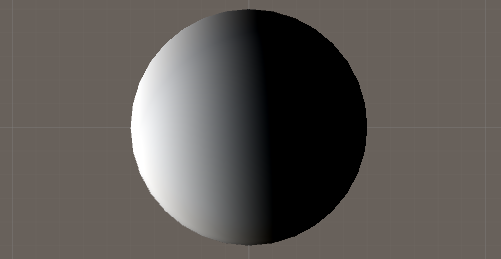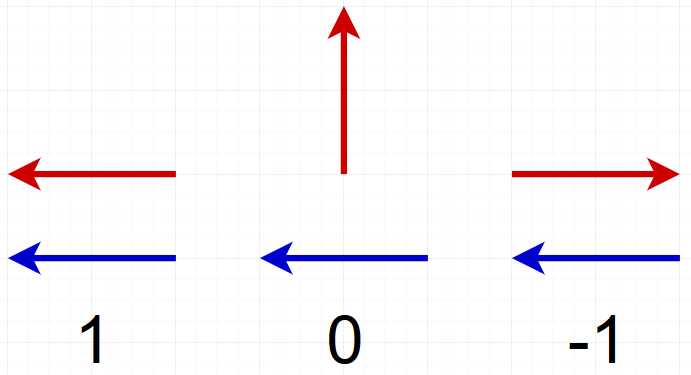First we will just get the dot product of the normal and a static vector so see better how it works. We then write the result into the emission channel, so we can see it well.

``````//the surface shader function which sets parameters the lighting function then uses
void surf (Input i, inout SurfaceOutputStandard o) {

//...

//get the dot product between the normal and the direction
float fresnel = dot(i.worldNormal, float3(0, 1, 0));
//apply the fresnel value to the emission
o.Emission = _Emission + fresnel;
}
``````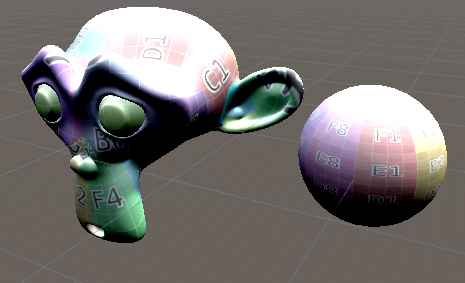We can now see that the surface gets brighter where it points up and darker where it points down. To prevent weird results with negative emission, we can clamp the fresnel value to the values between 0 to 1 before using it. For that we’ll use the saturate method. It does the same as a clamp from 0 to 1, but is faster on some GPUs. With that change we see how the fresnel only effects the top of our objects.

``````//the surface shader function which sets parameters the lighting function then uses
void surf (Input i, inout SurfaceOutputStandard o) {

//...

//get the dot product between the normal and the direction
float fresnel = dot(i.worldNormal, float3(0, 1, 0));
//clamp the value between 0 and 1 so we don't get dark artefacts at the backside
fresnel = saturate(fresnel);
//apply the fresnel value to the emission
o.Emission = _Emission + fresnel;
}
``````## Highlighting the outer Parts

The next step is to make the effect relative to our view direction instead of a fixed direction. In surface shaders we get the view direction by just adding it to our input struct.

If you’re making a unlit fresnel shader, you can get the view direction by subtracting the camera position from the world position of your vertex (I explain how to get the worldspace position in my planar mapping tutorial and you can get the camera position from the builtin variable _WorldSpaceCameraPos (you can just use it in your code without adding anything else)).

``````//input struct which is automatically filled by unity
struct Input {
float2 uv_MainTex;
float3 worldNormal;
float3 viewDir;
INTERNAL_DATA
};

//the surface shader function which sets parameters the lighting function then uses
void surf (Input i, inout SurfaceOutputStandard o) {

//...

//just apply the values for metalness and smoothness
o.Metallic = _Metallic;
o.Smoothness = _Smoothness;

//get the dot product between the normal and the view direction
float fresnel = dot(i.worldNormal, i.viewDir);
//clamp the value between 0 and 1 so we don't get dark artefacts at the backside
fresnel = saturate(fresnel);
//apply the fresnel value to the emission
o.Emission = _Emission + fresnel;
}
``````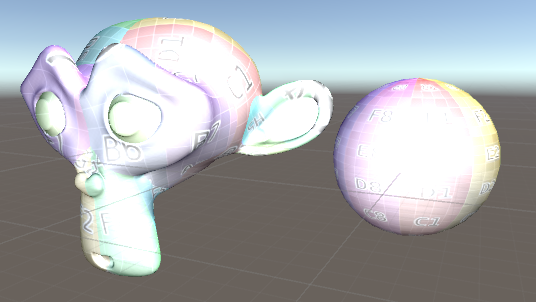That’s already working pretty well, but instead of the outside of the material, it’s illuminating the center of it. To invert that, we simply subtract if from 1, so the bright areas in this version don’t affect the color any more and the unaffected parts get highlighted.

``````//the surface shader function which sets parameters the lighting function then uses
void surf (Input i, inout SurfaceOutputStandard o) {

//...

//get the dot product between the normal and the view direction
float fresnel = dot(i.worldNormal, i.viewDir);
//invert the fresnel so the big values are on the outside
fresnel = saturate(1 - fresnel);
//apply the fresnel value to the emission
o.Emission = _Emission + fresnel;
}
``````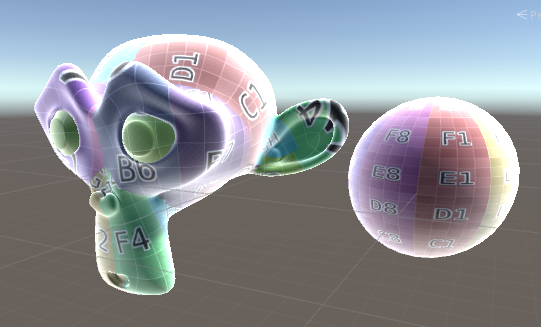## Add Fresnel Color and Intensity

To finish this shader I’d like to add some customisation options. First we add a fresnel color. For that we need a property and a value for that and then multiply our fresnel value with that color.

``````//...

_FresnelColor ("Fresnel Color", Color) = (1,1,1,1)

//...

float3 _FresnelColor;

//...

//the surface shader function which sets parameters the lighting function then uses
void surf (Input i, inout SurfaceOutputStandard o) {

//...

//get the dot product between the normal and the view direction
float fresnel = dot(i.worldNormal, i.viewDir);
//invert the fresnel so the big values are on the outside
fresnel = saturate(1 - fresnel);
//combine the fresnel value with a color
float3 fresnelColor = fresnel * _FresnelColor;
//apply the fresnel value to the emission
o.Emission = _Emission + fresnelColor;
}

//...
``````Next we’ll add a possibility to make the fresnel effect stronger or weaker by adding a exponent to it. We also add the powerslider attribute to the property of the exponent. That way values closer to 0 take up more space on the slider and can be adjusted more accurately (in this example the part of the slider from 0.25 to 1 is almost as big as the part from 1 to 4).

Exponents are pretty expensive so if you find a way to adjust your fresnel that fits you just as well, it’s probably better to switch to that, but exponents are also easy and nice to use.

``````
//...

[PowerSlider(4)] _FresnelExponent ("Fresnel Exponent", Range(0.25, 4)) = 1

//...

float _FresnelExponent;

//...

//the surface shader function which sets parameters the lighting function then uses
void surf (Input i, inout SurfaceOutputStandard o) {

//...

//get the dot product between the normal and the view direction
float fresnel = dot(i.worldNormal, i.viewDir);
//invert the fresnel so the big values are on the outside
fresnel = saturate(1 - fresnel);
//combine the fresnel value with a color
float3 fresnelColor = fresnel * _FresnelColor;
//raise the fresnel value to the exponents power to be able to adjust it
fresnel = pow(fresnel, _FresnelExponent);
//apply the fresnel value to the emission
o.Emission = _Emission + fresnelColor;
}

//...
``````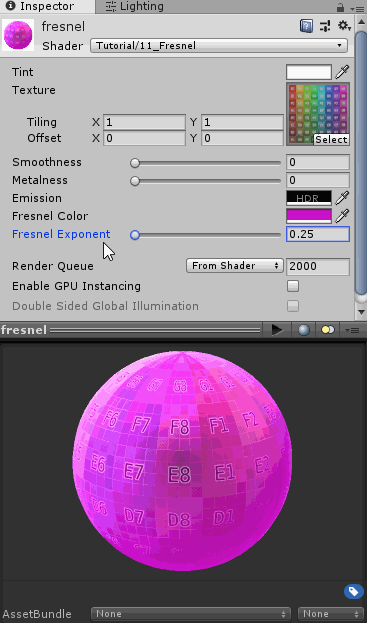A fresnel effect can also be used to fade textures or other effects among other things, but that’s for you to explore or maybe another tutorial.

``````Shader "Tutorial/012_Fresnel" {
//show values to edit in inspector
Properties {
_Color ("Tint", Color) = (0, 0, 0, 1)
_MainTex ("Texture", 2D) = "white" {}
_Smoothness ("Smoothness", Range(0, 1)) = 0
_Metallic ("Metalness", Range(0, 1)) = 0
[HDR] _Emission ("Emission", color) = (0,0,0)

_FresnelColor ("Fresnel Color", Color) = (1,1,1,1)
[PowerSlider(4)] _FresnelExponent ("Fresnel Exponent", Range(0.25, 4)) = 1
}
//the material is completely non-transparent and is rendered at the same time as the other opaque geometry
Tags{ "RenderType"="Opaque" "Queue"="Geometry"}

CGPROGRAM

//the shader is a surface shader, meaning that it will be extended by unity in the background to have fancy lighting and other features
//our surface shader function is called surf and we use the standard lighting model, which means PBR lighting
#pragma target 3.0

sampler2D _MainTex;
fixed4 _Color;

half _Smoothness;
half _Metallic;
half3 _Emission;

float3 _FresnelColor;
float _FresnelExponent;

//input struct which is automatically filled by unity
struct Input {
float2 uv_MainTex;
float3 worldNormal;
float3 viewDir;
INTERNAL_DATA
};

//the surface shader function which sets parameters the lighting function then uses
void surf (Input i, inout SurfaceOutputStandard o) {
//sample and tint albedo texture
fixed4 col = tex2D(_MainTex, i.uv_MainTex);
col *= _Color;
o.Albedo = col.rgb;

//just apply the values for metalness and smoothness
o.Metallic = _Metallic;
o.Smoothness = _Smoothness;

//get the dot product between the normal and the view direction
float fresnel = dot(i.worldNormal, i.viewDir);
//invert the fresnel so the big values are on the outside
fresnel = saturate(1 - fresnel);
//raise the fresnel value to the exponents power to be able to adjust it
fresnel = pow(fresnel, _FresnelExponent);
//combine the fresnel value with a color
float3 fresnelColor = fresnel * _FresnelColor;
//apply the fresnel value to the emission
o.Emission = _Emission + fresnelColor;
}
ENDCG
}
FallBack "Standard"
}
``````

I hope I could help you understand how fresnel effects work and you can use them for your own shaders if you want to.